﻿ 一类含周期激励Duffing系统的数值模拟

# 一类含周期激励Duffing系统的数值模拟Numerical Simulation of a Class of Duffing Systems with Periodic Excitation

Abstract: This paper mainly explores a Duffing system with periodic excitation. Based on Melnikov method, Euler discretization, fourth-order Runge-Kutta algorithm theory and Matlab program, it theoretically analyzes the dynamic phenomena of the system under different parameters, such as bifurcation, periodic motion and chaos, makes time series diagram, phase diagram, bifurcation diagram and maximum Lyapunov index diagram, and observes Duffing system when amplitude, frequency and damping terms change. Numerical simulation shows that when the excitation amplitude and frequency are constant, with the increase of damping term, the chaotic behavior of the system will change significantly, and the chaotic state will gradually disappear to form periodic motion, while when the influence of amplitude is considered, the chaotic state of the system will not change much.

1. 引言

2. Duffing系统的混沌机理及离散化

2.1. 考虑非线性Duffing系统

${x}^{″}+2\mu \alpha {x}^{\prime }+mx-n{x}^{3}=\mu f\mathrm{cos}\Omega t$ (1)

$\begin{array}{l}{x}^{\prime }=y\\ {y}^{\prime }=-2\mu \alpha y-mx+n{x}^{3}+\mu f\mathrm{cos}\Omega t\end{array}$ (2)

$\mu =0$ 时，考虑 $m=n=1$，上式的哈密顿系统对应的函数如下：

$H\left(x,y\right)=-\frac{1}{4}{x}^{4}+\frac{1}{2}{x}^{2}+\frac{1}{2}{y}^{2}$ (3)

${x}_{±}^{0}\left(t\right)=±\mathrm{tanh}\left(0.5\sqrt{2}t\right);{y}_{±}^{0}\left(t\right)=±0.5\sqrt{2}{\text{sech}}^{2}\left(0.5\sqrt{2}t\right)$ (4)

$\begin{array}{l}{M}_{±}\left({t}_{0}\right)={\int }_{-\infty }^{+\infty }{y}_{±}^{0}\left(t\right)\cdot \left[-2\alpha {y}_{±}^{0}\left(t\right)+f\mathrm{cos}\Omega \left(t-{t}_{0}\right)\right]\text{d}t\\ =-2\alpha {\int }_{-\infty }^{+\infty }{\left({y}_{±}^{0}\left(t\right)\right)}^{2}\text{d}t+f{\int }_{-\infty }^{+\infty }{y}_{±}^{0}\left(t\right)\mathrm{cos}\Omega \left(t-{t}_{0}\right)\\ =A\alpha +f{\int }_{-\infty }^{+\infty }{y}_{±}^{0}\left(t\right)\left(\mathrm{cos}\Omega t\mathrm{cos}\Omega {t}_{0}+\mathrm{sin}\Omega t\mathrm{sin}\Omega {t}_{0}\right)\\ =A\alpha +B\cdot f\mathrm{cos}\Omega {t}_{0}\end{array}$ (5)

$\frac{f}{\alpha }>\frac{2\sqrt{2}}{3}\cdot \frac{1}{\sqrt{2}\pi \Omega \text{csch}\left(\text{0}\text{.5}\sqrt{2}\pi \Omega \right)}$，存在 ${t}_{0}^{±}$，使得 ${M}_{±}\left({t}_{0}^{±}\right)=0$，又因为 ${M}_{±}\left({t}_{0}^{±}\right)=-\Omega fB\mathrm{sin}\left(\Omega {t}_{0}^{±}\right)\ne 0$，所以 ${M}_{±}\left({t}_{0}\right)$ 存在单重简单零点，则会产生Smale马蹄意义下的混沌现象。其中 $\frac{A}{B}=\frac{2}{3\pi \Omega }\mathrm{sinh}\left(0.5\sqrt{2}\pi \Omega \right)$，因此，当外激励振幅f与 $\alpha$ 之比超过Melnikov阈值 $\frac{A}{B}$ 时，Duffing系统会产生混沌现象。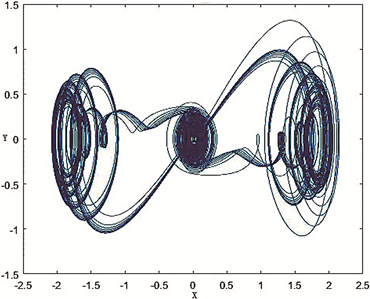Figure 1. System shows phase diagram and time series diagram when $\mu =0.1,\text{\hspace{0.17em}}f=0.35,\text{\hspace{0.17em}}\alpha =0.7,\text{\hspace{0.17em}}\Omega =0.1$

2.2. 对非线性Duffing系统离散化

$\begin{array}{l}{x}_{n+1}={x}_{n}+\Delta t{y}_{n}\\ {y}_{n+1}=-m\Delta t{x}_{n}+\left(1-2\mu \alpha \Delta t\right){y}_{n}+n{\left(\Delta t\right)}^{3}{x}_{n}^{3}+\Delta tp\end{array}$ (6)

${k}_{n}={x}_{n}+\Delta t{y}_{n}$，方程(6)变为：

$\begin{array}{l}{x}_{n+1}={k}_{n}\\ {k}_{n+1}=2\left(1-\mu \alpha \Delta t\right){k}_{n}+\left(2\mu \alpha \Delta t-m\Delta {t}^{2}-1\right){x}_{n}+n\Delta {t}^{4}{x}_{n}^{3}+\Delta {t}^{2}p\end{array}$ (7)

$\begin{array}{l}{x}_{n+1}={y}_{n}\\ {y}_{n+1}={\delta }_{1}{x}_{n}+{\delta }_{2}{x}_{n}^{3}+{\delta }_{3}{y}_{n}+p\end{array}$ (8)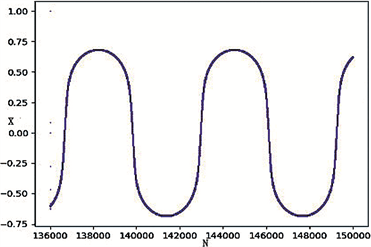Figure 2. Bifurcation diagram and time series diagram of system with respect to parameter p when ${\delta }_{1}=1.38,{\delta }_{2}=1.2,{\delta }_{3}=0.0035$Figure 3. Bifurcation diagram and time series diagram of system with respect to parameter p when ${\delta }_{1}=1.85,{\delta }_{2}=1.2,{\delta }_{3}=0.0035$

3. 四阶Runge-Kutta算法理论过程及仿真计算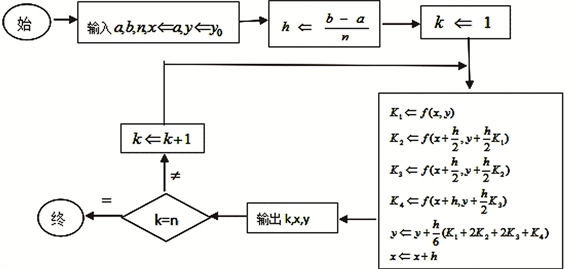Figure 4. Algorithm implementation

1) 输入 $a,b$ 区间等分数n，初值 ${y}_{0}$。2) 导出 $y\left(x\right)$ 在x的n个点处近似解析值y。3) 设置 $h=\frac{b-a}{n},k=0,x=a,y={y}_{0}$。4) 计算 ${K}_{1}=f\left(x,y\right)$${K}_{2}=f\left(x+\frac{h}{2},y+\frac{h}{2}{K}_{1}\right)$${K}_{3}=f\left(x+\frac{h}{2},y+\frac{h}{2}{K}_{2}\right)$${K}_{4}=f\left(x+h,y+h{K}_{3}\right)$，置 $y+\frac{h}{6}\left({K}_{1}+2{K}_{2}+2{K}_{3}+{K}_{4}\right)⇒y,x+h⇒x$，输出(见图4)。

① 通过实际工程模型规律，依据相关公式和定理得出相应微分方程和对应的初始条件。

② 运用相关数值变量替换，将高阶的方程变为一阶的微分方程组，并写出相应初始值。

③ 编写M文件，调整命令数据，运行得到指定区间的数值解。

$\begin{array}{l}{{y}^{\prime }}_{1}={y}_{2}\\ {{y}^{\prime }}_{2}=-m{y}_{1}+3n{x}_{1}^{2}{y}_{1}-2\mu \alpha {y}_{2}\end{array}$ (9)

$A=\left(\begin{array}{cc}0& 1\\ -m& -2\mu \alpha \end{array}\right),F\left(t\right)=\left(\begin{array}{cc}0& 0\\ -3n{x}_{1}^{2}& 0\end{array}\right),Y=\left(\begin{array}{c}{y}_{1}\\ {y}_{2}\end{array}\right)$ 方程(9)变为：

${Y}^{\prime }=\left[A+F\left(t\right)\right]\cdot Y$ (10)

$\lambda =\underset{x\to \infty }{\mathrm{lim}}\frac{1}{t}\mathrm{log}‖\frac{Y\left(t\right)}{Y\left(0\right)}‖,‖Y\left(t\right)‖=\sqrt{{y}_{1}^{2}+{y}_{2}^{2}}$ (11)

$\lambda =\underset{N\to \infty }{\mathrm{lim}}\frac{1}{N\Delta t}\underset{i=1}{\overset{N}{\sum }}{\int }_{{t}_{i-1}}^{{t}_{i}}\frac{{M}^{\prime }\left(\tau \right)}{M\left(\tau \right)}\text{d}\tau$ (12)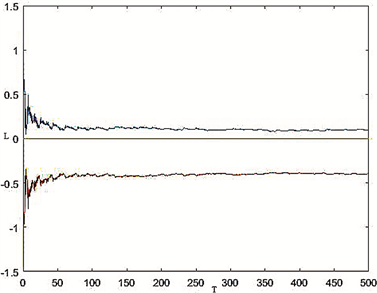Figure 5. Maximum lyapunov exponent of the system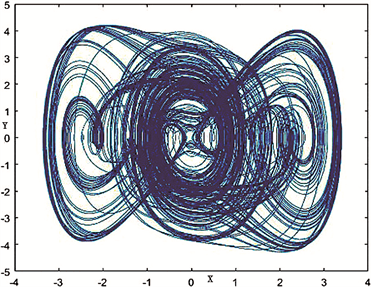Figure 6. System shows phase diagram and time series diagram when $\Omega =0.7,\text{\hspace{0.17em}}\mu f=0.1,\text{\hspace{0.17em}}\mu \alpha =0.15$

4. 周期激励的振幅对Duffing系统的影响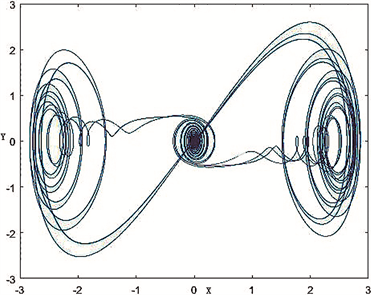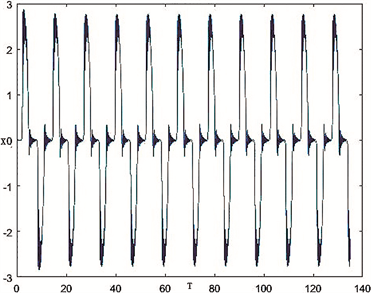(a)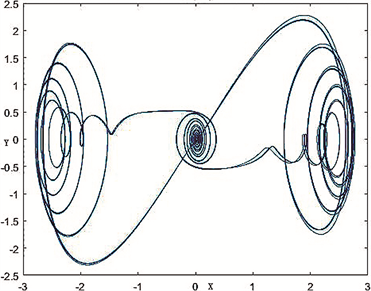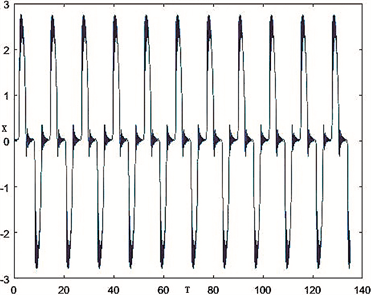(b)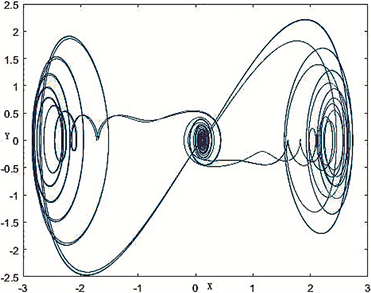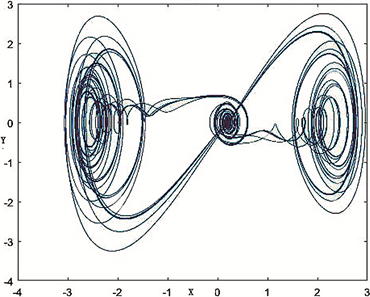(c)

Figure 7. (a) and (b) show the system in-time phase diagram and time series diagram, and (c) shows the system in-time phase diagram

5. 阻尼项对Duffing系统的影响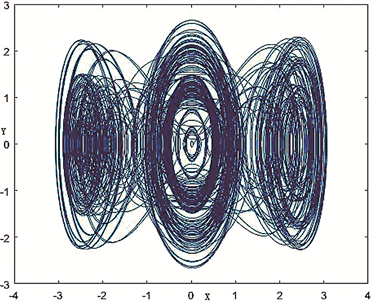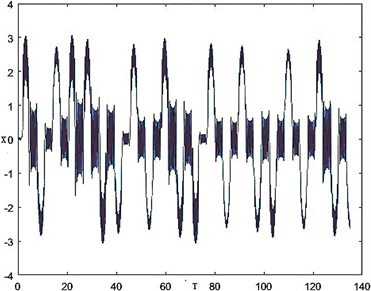(a)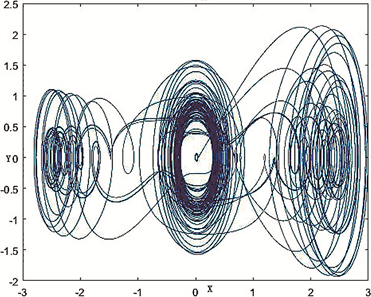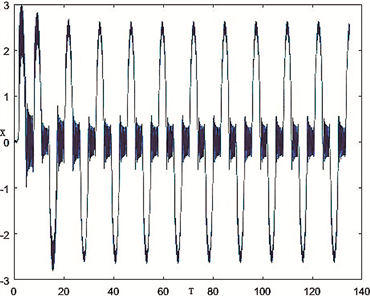(b)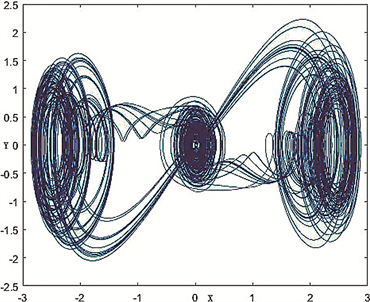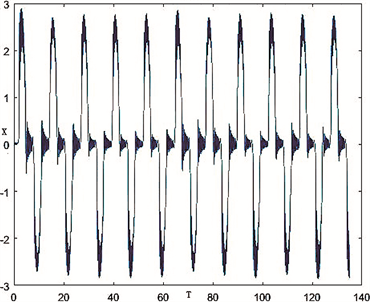(c)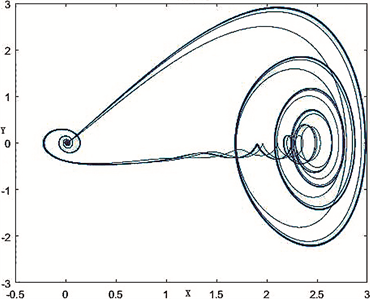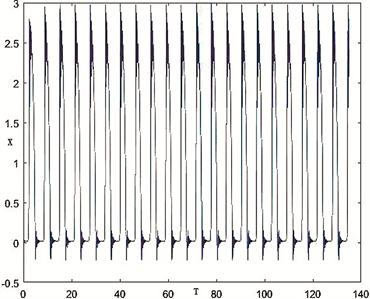(d)

Figure 8. (a), (b), (c) and (d) show the time system phase diagram and time series diagram when ${k}_{1}=0.01,\text{\hspace{0.17em}}{k}_{1}=0.05,\text{\hspace{0.17em}}{k}_{1}=0.15,\text{\hspace{0.17em}}{k}_{1}=0.5$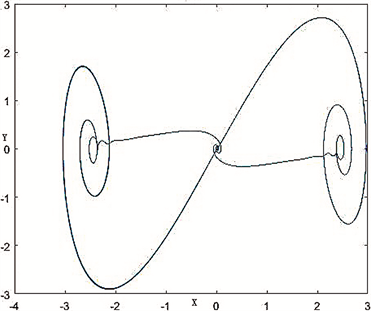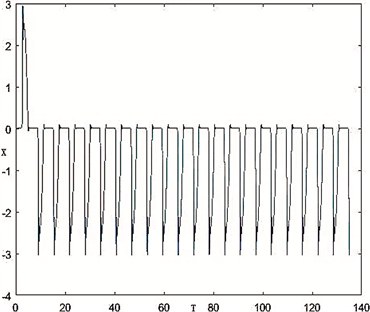(a)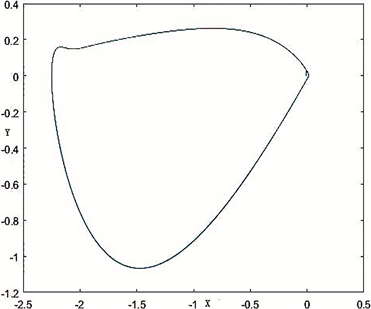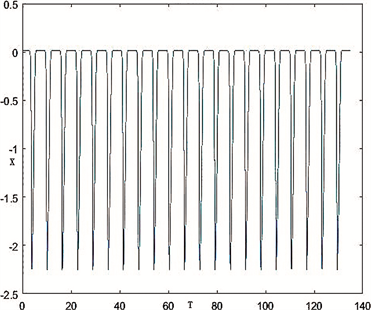(b)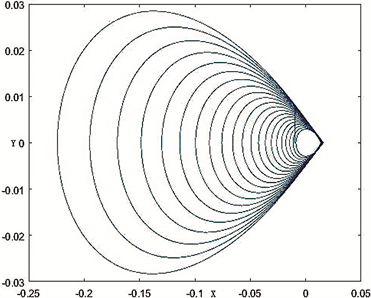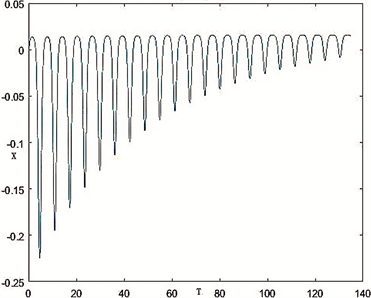(c)

Figure 9. (a), (b), (c) show the time system phase diagram and time series diagram when ${k}_{1}=1.2,\text{\hspace{0.17em}}{k}_{1}=4.5,\text{\hspace{0.17em}}{k}_{1}=20$

6. 周期激励的频率对Duffing系统的影响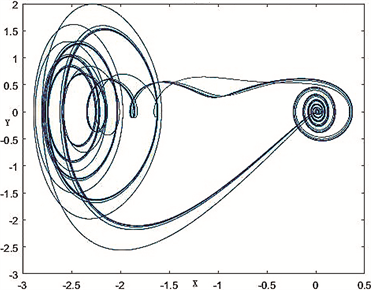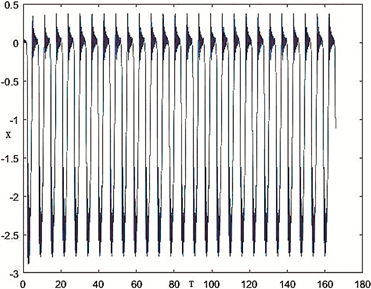(a)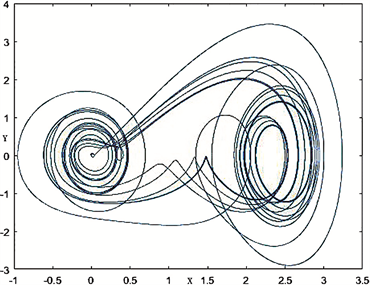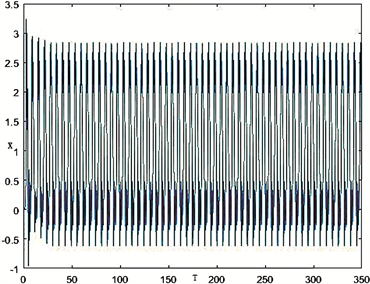(b)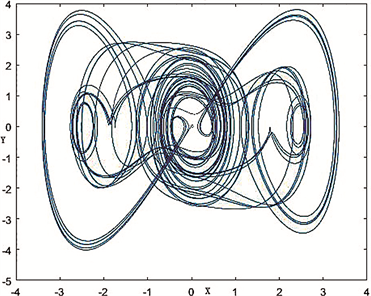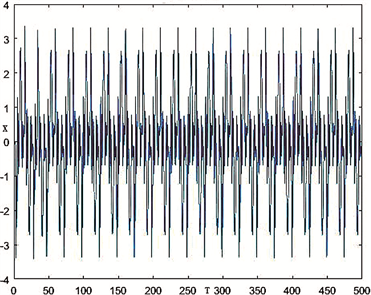(c)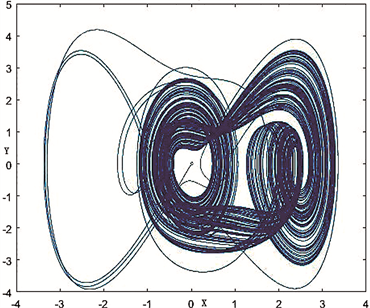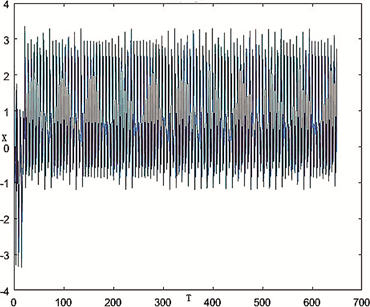(d) Figure10. (a), (b), (c), (d) show the time phase diagram and time series diagram respectively when $\Omega =0.1655,\text{\hspace{0.17em}}\Omega =0.35,$ $\text{\hspace{0.17em}}\Omega =0.5,\text{\hspace{0.17em}}\Omega =0.65$图10. (a)、(b)、(c)、(d)表示当 $\Omega =0.1655,\text{\hspace{0.17em}}\Omega =0.35,\text{\hspace{0.17em}}\Omega =0.5,\text{\hspace{0.17em}}\Omega =0.65$ 时相图和时间序列图现不妨固定 $\alpha =1.5$，则设定参数 ${k}_{1}=0.15,\text{\hspace{0.17em}}{k}_{2}=0.1$，并保持系统其它状态参数不变，分别取 $\Omega =0.1655,\text{\hspace{0.17em}}\Omega =0.35,\text{\hspace{0.17em}}\Omega =0.5,\text{\hspace{0.17em}}\Omega =0.65$，作出系统的时间序列图和相图。如图10(a)~(d)所示，当 $\Omega =0.1655$ 时，系统出现单独左支的混沌吸引子，而当 $\Omega$ 增大到0.35时，系统出现右支的混沌吸引子，随后当 $\Omega =0.5$ 时，出现两个混沌吸引子共存在状态，但当 $\Omega$ 增大到0.65时，混沌吸引集少量吸引在左支，大量吸引在右支情况。从计算 $\Omega =0.1655$ 处的最大Lyapunov指数 $\lambda =0.813594$ 为正表明这是混沌状态，同时从时间序列图也可发现系统随时间运动的区域以及变化程度，因此频率逐渐增大会影响其混沌情况。若将 $\Omega$ 略微减小，不妨取 $\Omega =0.1355,\text{\hspace{0.17em}}\Omega =0.08,\text{\hspace{0.17em}}\Omega =0.05$ 时研究它对系统产生的影响。通过观测图11(a)~(c)的相图和时间序列图可知，系统由单独左支的情况变为左右两支共存情况，即两混沌吸引子共存，而后在初始值附近又出现单独左支情形，然而当 $\Omega$ 继续变小时又重新出现左右两支共存现象，此时仍为混沌状态，但与此前的混沌吸引子共存状态稍有些不同，而具体情况有待进一步研究。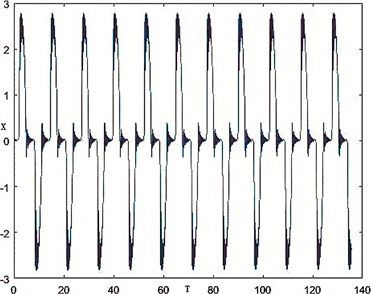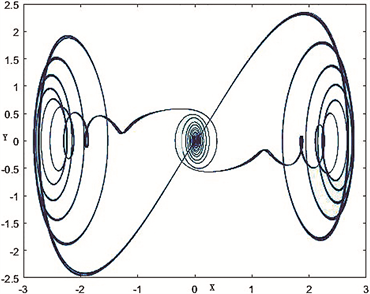(a)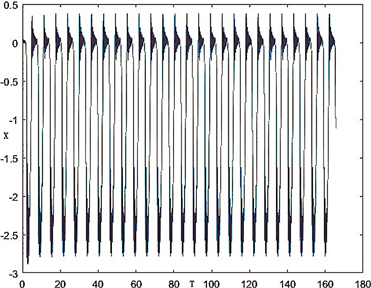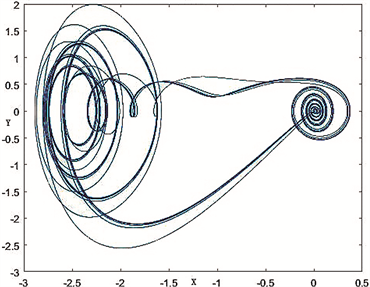(b)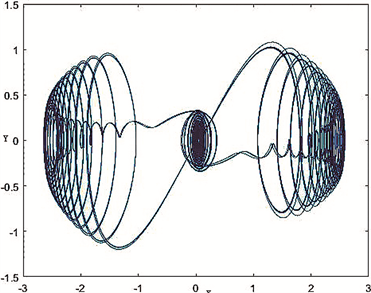(c)

Figure 11. (a), (b), (c) show the time phase diagram and time series diagram respectively when $\Omega =0.1355,\text{\hspace{0.17em}}\Omega =0.08,$ $\Omega =0.05$

(c)

7. 结论

 Li, M.D., Yuan, Z.C. and Luo, D.B. (2020) In-Depth Study of Chaos in a Nonlinear Dynamic System. University Physics, 39, 33-37.

 Li, Q.D. and Yang, X.S. (2012) Progresses on Chaotic Dynamics Study with Topological Horseshoes. Journal of Dynamics & Control, 4, 293-298.

 Cai, M.X., Yang, J.P. and Deng, J. (2014) Bifurcations and Chaos in Duffing Equation with Damping and External Excitations. Acta Mathematicae Applicatae Sinica, English Series, 30, 483-504.
https://doi.org/10.1007/s10255-014-0284-0

 Fernando, A., Ana, P.S.D. and Carla, M.A.P. (2010) Quasi-Periodic States in Coupled Rings of Cells. Communications in Nonlinear Science and Numerical Simulation, 15, 1048-1062.
https://doi.org/10.1016/j.cnsns.2009.05.035

 Tao, J. and Zhi, Y.Y. (2017) Bifurcations and Chaos in the Duffing Equation with Parametric Excitation and Single External Forcing. International Journal of Bifurcation & Chaos, 27, Article ID: 1750125.
https://doi.org/10.1142/S0218127417501255

 Plaut, R.H. and Hsich, J.C. (1987) Chaos in a Mechanism with Time Delays under Parametric and External Excitation. Journal of Sound and Vibration, 114, 73-90.
https://doi.org/10.1016/S0022-460X(87)80235-3

 Raghothama, A. and Narayanan, S. (2002) Periodic Response and Chaos in Nonlinear Systems with Parametric Excitation and Time Delay. Nonlinear Dynamics, 27, 341-365.
https://doi.org/10.1023/A:1015207726565

 刘延柱, 陈立群. 非线性振动[M]. 北京: 高等教育出版社, 2011: 224-228.

 Zuo, Z.L. and Yu, X. (2019) A Design Method of Chaotic Synchronous Multi-Stable Manifold. Materials Science and Engineering, 544, 12-37.
https://doi.org/10.1088/1757-899X/544/1/012037

 He, H.J., Cui, Y. and Sun, G. (2019) Dynamic Analysis and Chaos Control of a New Nonlinear System. Journal of Jilin University, 57, 1224-1230.

 李庆, 关治, 白峰山. 数值计算原理[M]. 北京: 清华大学出版社, 2004.

 Huang, X.-R. and Jézéquel, L.B. (2018) Nonlinear Modal Synthesis for Analyzing Structures with a Frictional Interface Using a Generalized Masing Model. Journal of Sound & Vibration, 434, 166-191.
https://doi.org/10.1016/j.jsv.2018.07.027

 Qian, Y.H. and Yan, D.M. (2018) Fast-Slow Dynamics Analysis of a Coupled Duffing System with Periodic Excitation. International Journal of Bifurcation & Chaos in Applied Sciences & Engineering, 28, Article ID: 1850148.
https://doi.org/10.1142/S0218127418501481

 Zheng, J.K., Zhang, X.F. and Bi, Q.S. (2019) Clusters Oscillation and Delayed Fork Bifurcation in a Class of Chaotic Systems. Chinese Journal of Theoretical and Applied Mechanics, 51, 540-549.

 Cai, M.X. and Yang, J.P. (2006) Bifurcation of Periodic Orbits and Chaos in Duffing Equation. Acta Mathematicae Applicatae Sinica, English Series, 22, 495-508.
https://doi.org/10.1007/s10255-006-0325-4

 Ji, J.C. and Leung, A.Y. (2002) Bifurcation Control of a Parametrically Excited Duffing System. Nonlinear Dynamics, 27, 411-417.
https://doi.org/10.1023/A:1015221422293

 Gong, S. and Wang, X.Yu. (2019) Dynamic Analysis of Vibrating Flip-Flow Screen Based on a Nonlinear Model of Shear Spring. Journal of the China Coal Society, 44, 3241-3249.

 Chen, Y.N., Meng, W.J. and Qian, Y.H. (2020) Fixed Point Chaos and Fold/Fold Bursting of a Class of Duffing Systems and the Mechanism Analysis. Chinese Journal of Theoretical and Applied Mechanics, 52, 1475-1484.

 Li, X.H., et al. (2019) New Periodic-Chaotic Attractors in Slow-Fast Duffing System with Periodic Parametric Excitation. Scientific Reports, 9, Article No. 11185.
https://doi.org/10.1038/s41598-019-46768-7

Top